# Mathematical problems for self assessment

###### SECOND ORDER ORDINARY DIFFERENTIAL EQUATIONS

1. Find the complementary function (and thus the general solution) of the following homogeneous second order linear differential equations:

(i)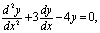(ii)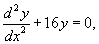(iii)(iv)(v)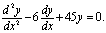[Ans. (i), (ii), (iii)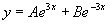, (iv)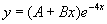, (v)].

2. Find the particular solution of the following homogeneous second order linear differential equations:

(i)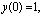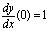,

(ii)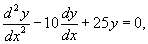.

[Ans. (i), (ii)].

3. Find the general solution of the inhomogeneous second order linear differential equation,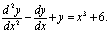[Ans.].

4. Find the general solution of the inhomogeneous second order linear differential equation,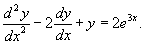[Ans.].

5. Find the particular solution of the inhomogeneous second order linear differential equation,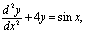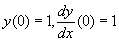.

[Ans.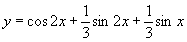].

6. Find the general solution of the inhomogeneous second order linear differential equation,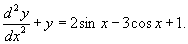[Ans.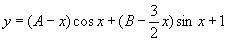].

7. Find the particular solution of the inhomogeneous second order linear differential equation,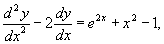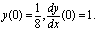[Ans.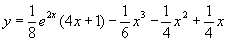].

8. The charge, q, on a capacitor in an LCR circuit satisfies the second order differential equation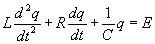,

where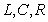and E are constants. Show that ifthen the general solution of the this equation is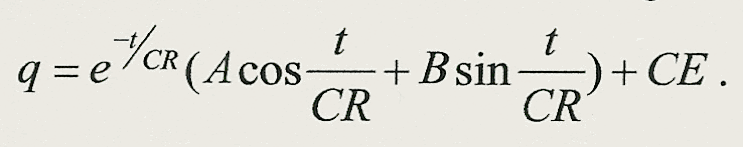If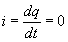and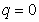when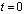show that the instantaneous current in the circuit is given by9. The displacement, x, of a periodically forced undamped harmonic oscillator satisfies the second order differential equation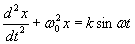where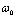is the undamped natural frequency of the oscillator and k and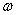are the amplitude and frequency respectively of the periodic force applied. Suppose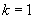, then find the general solution to this equation in the cases:

(i),

(ii)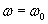.

In case (ii) what happens to the oscillator as time t increases?

[Ans. (i)(ii)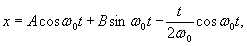as t increases the displacement x evolves linearly with t and resonance occurs.].